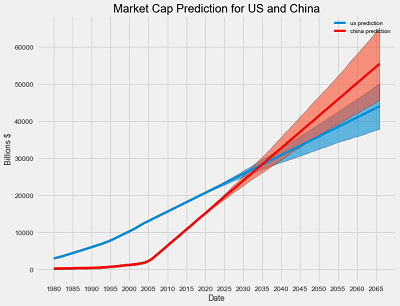Auto ByteScience AI# 利用进化方法自动生成神经网络：深度进化网络DENSER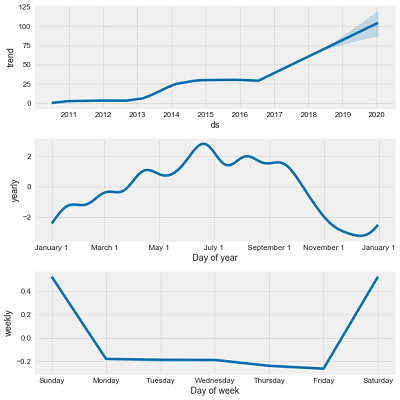pip install quandl

Quandl 是免费的，你可以每天提出 50 个访问请求而无需注册。如果注册一个免费的帐户，你会得到一个 API 密钥，允许无限制次数的请求。```# quandl for financial dataimport quandl
# pandas for data manipulation
import pandas as pdquandl.ApiConfig.api_key = 'getyourownkey!'
# Retrieve TSLA data from Quandltesla = quandl.get('WIKI/TSLA')
# Retrieve the GM data from Quandlgm = quandl.get('WIKI/GM')gm.head(5)```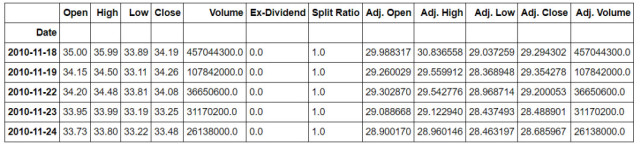GM（通用汽车）数据快照

Quandly 自动将数据放入 Pandas 数据框（DataFrame）中，DataFrame 是数据科学家的首选数据类型。（对于其他公司，只需用「TSLA」或「GM」替换股票代码，你也可以指定日期范围）

Pandas dataframe 可以很容易地用内置方法绘图：

`# The adjusted close accounts for stock splits, so that is what we should graphplt.plot(gm.index, gm['Adj. Close'])plt.title('GM Stock Price')plt.ylabel('Price (\$)');plt.show()plt.plot(tesla.index, tesla['Adj. Close'], 'r')plt.title('Tesla Stock Price')plt.ylabel('Price (\$)');plt.show();`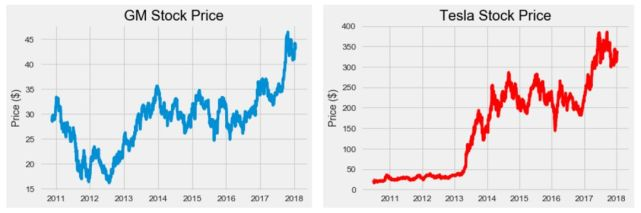`# Yearly average number of shares outstanding for Tesla and GMtesla_shares = {2018: 168e6, 2017: 162e6, 2016: 144e6, 2015: 128e6, 2014: 125e6, 2013: 119e6, 2012: 107e6, 2011: 100e6, 2010: 51e6}gm_shares = {2018: 1.42e9, 2017: 1.50e9, 2016: 1.54e9, 2015: 1.59e9, 2014: 1.61e9, 2013: 1.39e9, 2012: 1.57e9, 2011: 1.54e9, 2010:1.50e9}# Create a year columntesla['Year'] = tesla.index.year# Take Dates from index and move to Date columntesla.reset_index(level=0, inplace = True)tesla['cap'] = 0# Calculate market cap for all yearsfor i, year in enumerate(tesla['Year']): # Retrieve the shares for the year shares = tesla_shares.get(year) # Update the cap column to shares times the price tesla.ix[i, 'cap'] = shares * tesla.ix[i, 'Adj. Close']`

`# Merge the two datasets and rename the columnscars = gm.merge(tesla, how='inner', on='Date')cars.rename(columns={'cap_x': 'gm_cap', 'cap_y': 'tesla_cap'}, inplace=True)# Select only the relevant columnscars = cars.ix[:, ['Date', 'gm_cap', 'tesla_cap']]# Divide to get market cap in billions of dollarscars['gm_cap'] = cars['gm_cap'] / 1e9cars['tesla_cap'] = cars['tesla_cap'] / 1e9cars.head()`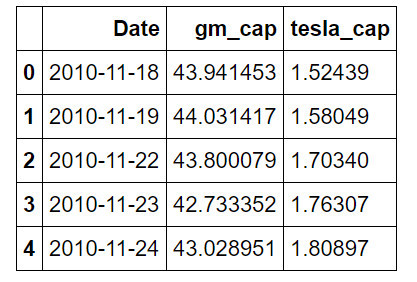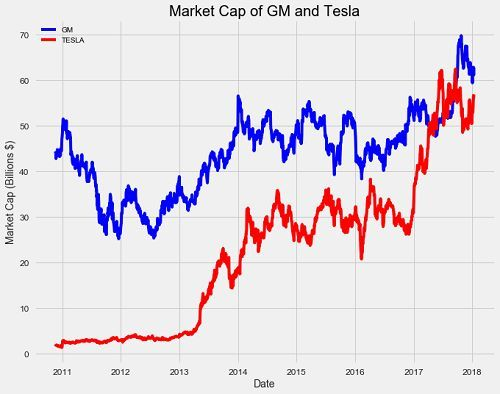`import numpy as np# Find the first and last time Tesla was valued higher than GMfirst_date = cars.ix[np.min(list(np.where(cars['tesla_cap'] > cars['gm_cap']))), 'Date']last_date = cars.ix[np.max(list(np.where(cars['tesla_cap'] > cars['gm_cap']))), 'Date']print("Tesla was valued higher than GM from {} to {}.".format(first_date.date(), last_date.date()))Tesla was valued higher than GM from 2017-04-10 to 2017-09-21.`

Facebook 于 2017 年发布基于 Python 和 R 的 Prophet 包，它极大地帮助了数据科学家的工作。Prophet 设计目的是用日常观测数据分析时间序列，这些数据在不同尺度衡量下具有模式规律。它同时对建模节日效应的时间序列和添加人工变化点（changepoint）有出色的能力，但在本文中我们将仅运用基本功能来建模和运行。

`import fbprophet# Prophet requires columns ds (Date) and y (value)gm = gm.rename(columns={'Date': 'ds', 'cap': 'y'})# Put market cap in billionsgm['y'] = gm['y'] / 1e9# Make the prophet model and fit on the datagm_prophet = fbprophet.Prophet(changepoint_prior_scale=0.15)gm_prophet.fit(gm)`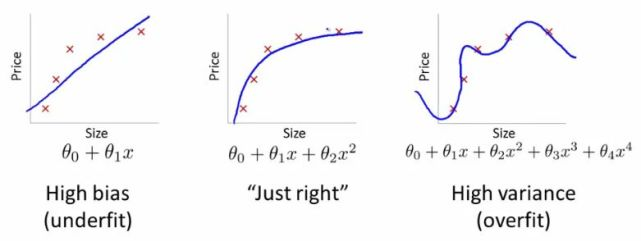`# Make a future dataframe for 2 yearsgm_forecast = gm_prophet.make_future_dataframe(periods=365 * 2, freq='D')# Make predictionsgm_forecast = gm_prophet.predict(gm_forecast)`

`gm_prophet.plot(gm_forecast, xlabel = 'Date', ylabel = 'Market Cap (billions \$)')plt.title('Market Cap of GM');`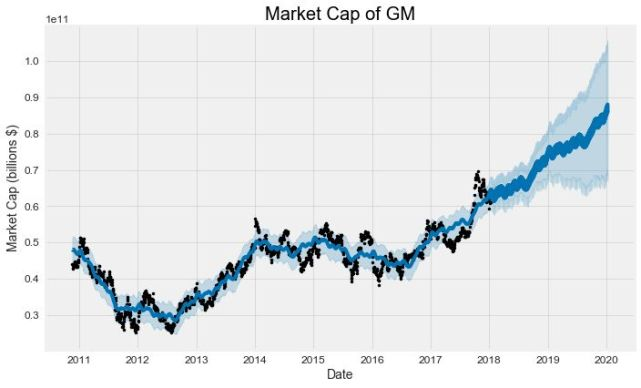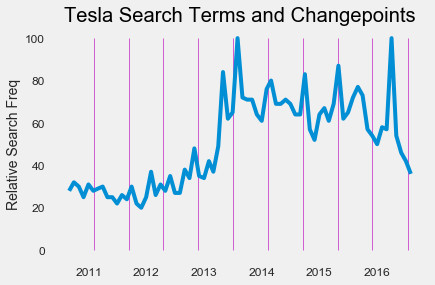`gm_names = ['gm_%s' % column for column in gm_forecast.columns]tesla_names = ['tesla_%s' % column for column in tesla_forecast.columns]# Dataframes to mergemerge_gm_forecast = gm_forecast.copy()merge_tesla_forecast = tesla_forecast.copy()# Rename the columnsmerge_gm_forecast.columns = gm_namesmerge_tesla_forecast.columns = tesla_names# Merge the two datasetsforecast = pd.merge(merge_gm_forecast, merge_tesla_forecast, how = 'inner', left_on = 'gm_ds', right_on = 'tesla_ds')# Rename date columnforecast = forecast.rename(columns={'gm_ds': 'Date'}).drop('tesla_ds', axis=1)`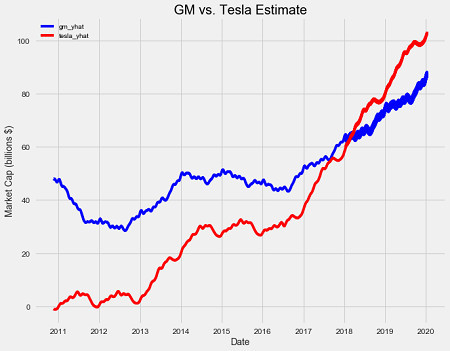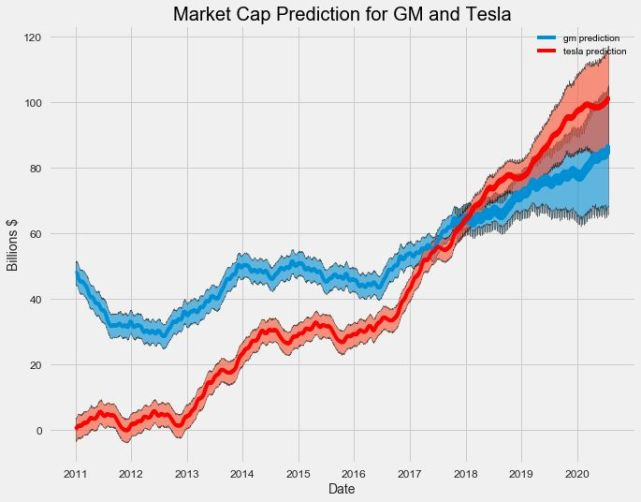`# Plot the trends and patternsgm_prophet.plot_components(gm_forecast)`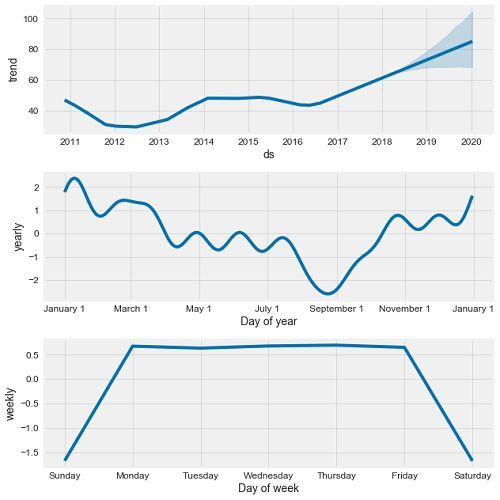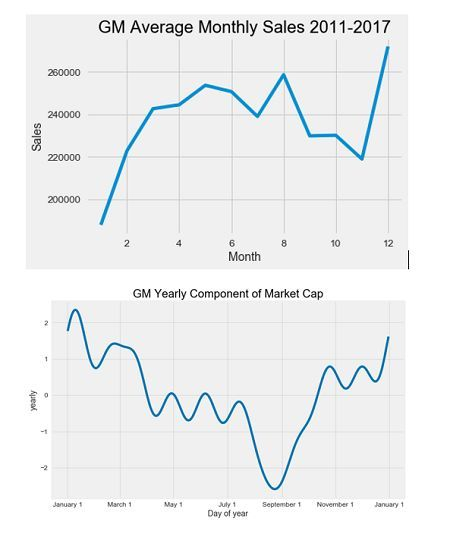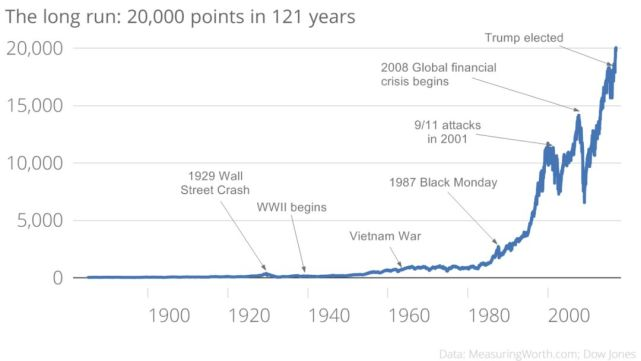Prophet 也可以应用于更大规模的数据测量，如国内生产总值（衡量一个国家经济总体规模）。我根据美国和中国的历史 GDP 创建了 prophet 模型并做了以下预测。